# Fill a grid with colour

#### 25 September 2014

The fourth post of the aftershock series is about a small function, fillgrid, with which you can easily plot a grid of filled rectangles.

## fillgrid()

Quite often I come across the situation that I have gridded data, where the grid-spacing is not necessarily regular, nor do all cells have a value associated (there are empty cells). To visualize these volumes I like to show either slices of it, or stack the volume in one dimension and plot it with intensity showing the number of cells. The function fillgrid is a customised wrapper for plt.fill to fill gridded data with colours from a colormap or with a single colour and transparency.

In :
import numpy as np
import matplotlib as mpl
import matplotlib.pyplot as plt
from IPython.display import Image
# Increase font size,
mpl.rc('font', size=16)
# Define colours (taken from http://colorbrewer2.org)
clr = ['#377eb8', '#e41a1c', '#4daf4a', '#984ea3', '#ff7f00', '#ffff33', '#a65628']


### Load the fillgrid-function

(You can find it in the notebook adashof.ipynb, in the same repo as this notebook).

In :
%load -s fillgrid adashof.py

In :
def fillgrid(xval, yval, values, style='colour', cmap=mpl.cm.Spectral,
unicol='#000000', lc='k', lw=0.5):
"""Fill rectangular grid with colours or a colour and transparency.

Parameters
----------
xval, yval : array
Grid-points in x- and in y-direction.
values : array, dimension: (x-1)-by-(y-1)
Values between 0 and 1
style : string, optional; {<'colour'>, 'alpha'}
Defines if values represent colour or alpha.
cmap : mpl.cm-element, optional
Colormap colours are chosen from; only used if style='colour'
unicol : HEX-colour
Colour used with transparency; only used if style='alpha'
lc, lw : optional
Line colour and width, as in standard plots.

Returns
-------
rct : list
List of plotted polygon patches.
"""

# Ravel values, and set NaN's to zero
rval = values.ravel()
rvalnan = np.isnan(rval)
rval[rvalnan] = 0

# Define colour depending on style
if style == 'alpha':
# Create RGB from HEX
unicol = mpl.colors.colorConverter.to_rgb(unicol)
# Repeat colour for all values,
# filling the value into the transparency column
colour = np.vstack((np.repeat(unicol, len(rval)).reshape(3, -1),
rval)).transpose()
else:
# Split cmap into 101 points from 0 to 1
cmcol = cmap(np.linspace(0, 1, 101))
# Map the values onto these
colour = cmcol[list(map(int, 100*rval))]
# Set transparency to 0 for NaN's
colour[rvalnan, -1] = 0

# Draw all rectangles at once
xxval = np.array([xval[:-1], xval[:-1], xval[1:], xval[1:]]).repeat(
len(yval)-1, axis=1).reshape(4, -1)
yyval = np.array([yval[:-1], yval[1:], yval[1:], yval[:-1]]).repeat(
len(xval)-1, axis=0).reshape(4, -1)
rct = mpl.pyplot.gca().fill(xxval, yyval, lw=lw, ec=lc)

# Map the colour onto a list
cls = list(map(mpl.colors.rgb2hex, colour))

# Adjust colour and transparency for all cells
for ind in range(len(rct)):
rct[ind].set_facecolor(cls[ind])
rct[ind].set_alpha(colour[ind, -1])

return rct


### Explanatory basic example

I show the use of fillgrid here with a small example. First we create some random coordinates for 20x10x3 cells (hence 21 x-coordinates, 11 y-coordinates, and 4 z-coordinates), where the cell-width in x- and y-directions is randomly 1, 2, or 3; it is always 1 in z-direction. We fill this volume with random numbers between 0 and 10, and roughly a third of the cells are NaN’s.

In :
# Create random coordinates
# X-direction 21, Y-direction 11, Z-direction 4
# X/Y-direction random spacing {1, 2, or 3}
x = np.cumsum(np.r_[0, np.random.randint(1, 4, 20)])
y = np.cumsum(np.r_[0, np.random.randint(1, 4, 10)])
z = np.arange(4)

# Create random values for cubes define by above coordinates
# Values are between 0 and 10, 1/3 are NaN's
maxval = 10
val = np.random.rand(len(x)-1, len(y)-1, len(z)-1)*maxval*1.5-maxval/2.
val[val<=0] = np.NaN

# Small figure-fct to create the example plots
def create_figure():
fig = plt.figure()
ax = plt.gca()
ax.set_axis_off()
ax.axis('equal')
ax.set_xlim([-.5, max(x)+.5])
ax.set_ylim([-.5, max(y)+.5])
return fig, ax

# Plot the colorbar, so we can associate colours with values
fig0 = plt.figure(figsize=(8, .3))
ax0 = fig0.add_axes([0.05, 0.05, 0.9, 0.9])
cb1 = mpl.colorbar.ColorbarBase(ax0, cmap=mpl.cm.Spectral, orientation='horizontal',
norm=mpl.cm.colors.Normalize(vmin=0, vmax=maxval))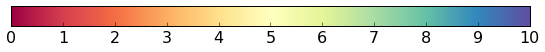Plotting coloured slices of this volume is now pretty straight forward with fillgrid:

1. Top slice with black lines

The Function fillgrid expects values between 0 and 1; we therefore divide (normalise) the values by the maximum value maxval.

In :
fig1, ax1 = create_figure() # figure fct as defined above
rct = fillgrid(x, y, val[:,:,0]/maxval, 'colour')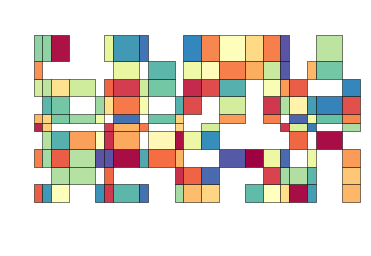2. Middle slice with white lines

In :
fig2, ax2 = create_figure()
rct = fillgrid(x, y, val[:,:,1]/maxval, 'colour', lc='w', lw=1)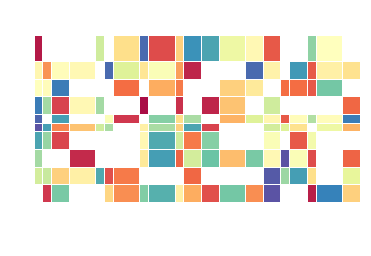3. Bottom slice, without lines and another colormap

In :
fig3, ax3 = create_figure()
rct = fillgrid(x, y, val[:,:,2]/maxval, 'colour', cmap=mpl.cm.PuOr, lc='none')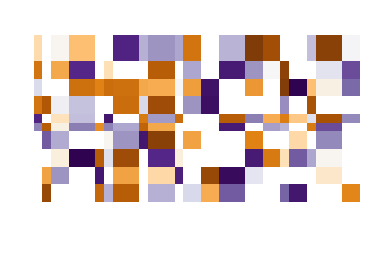Instead of plotting the values as colours I can also plot them as transparency. Here I stack the volume in z-direction, and plot the number of cells as transparencies of blue.

4. Stacked in z-direction, transparency reflects number of cells

In :
fig4, ax4 = create_figure()

# Calculate the accumulated cell-density (normalised stack)
# A. Create a copy of val of ones
alphaval = np.ones(np.shape(val))
# B. 1 where val>0 , else 0
alphaval[np.isnan(val)] = 0
# C. Sum and normalize
alphaval = alphaval.sum(axis=2)/(len(z)-1)

# Plot the result
rct = fillgrid(x, y, alphaval, style='alpha', unicol=clr, lc=clr, lw=2)### Bigger examples

The above is a small example with few cells to show the functionality of fillgrid. However, fillgrid works well on much larger data as well. Below are two figures out of my Ph.D. thesis, for which I used fillgrid.

The first example is a 3D reservoir model, which has over 100‘000 cells. I plotted this model as stacked versions in each direction, with the density shown by the transparency value. (The dashed lines refer to sections shown in the next figure in the thesis, which is not shown here.)

In :
Image(filename='data/gridfill/origreservoir.png')

Out: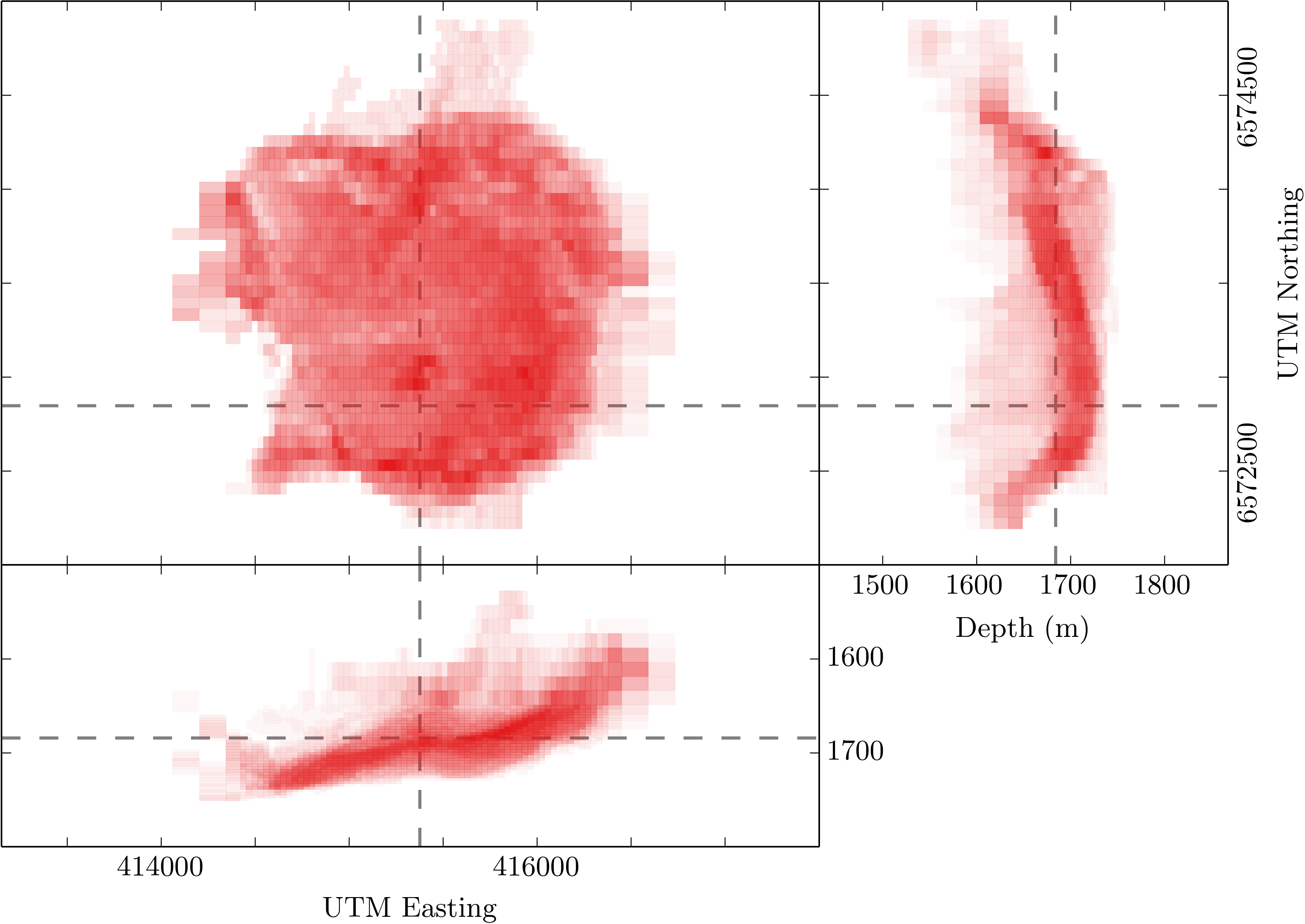The second example is a 2D section of a resistivity model which contains also thousands of cells.

In :
Image(filename='data/gridfill/fmgridcolor.png')

Out: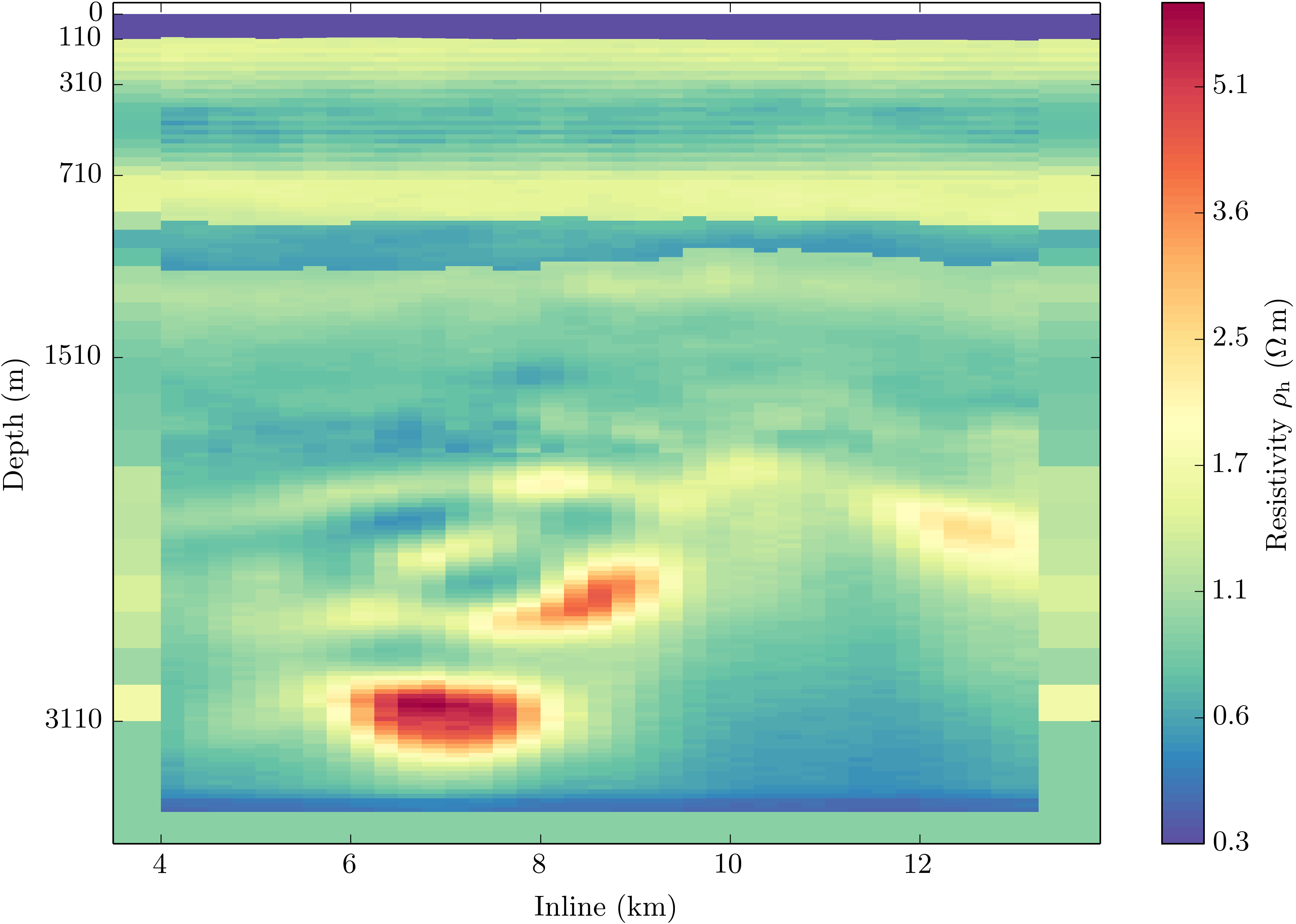The above notebook Fillgrid.ipynb can, as usual, be found on my GitHub page in the blog-notebooks-repo.